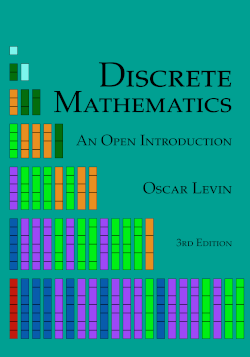# Discrete Mathematics: An Open Introduction, 3rd edition

## Chapter3Symbolic Logic and Proofs

Logic is the study of consequence. Given a few mathematical statements or facts, we would like to be able to draw some conclusions. For example, if I told you that a particular real-valued function was continuous on the interval $$[0,1]\text{,}$$ and $$f(0) = -1$$ and $$f(1) = 5\text{,}$$ can we conclude that there is some point between $$[0,1]$$ where the graph of the function crosses the $$x$$-axis? Yes, we can, thanks to the Intermediate Value Theorem from Calculus. Can we conclude that there is exactly one point? No. Whenever we find an “answer” in math, we really have a (perhaps hidden) argument. Mathematics is really about proving general statements (like the Intermediate Value Theorem), and this too is done via an argument, usually called a proof. We start with some given conditions, the premises of our argument, and from these we find a consequence of interest, our conclusion.
The problem is, as you no doubt know from arguing with friends, not all arguments are good arguments. A “bad” argument is one in which the conclusion does not follow from the premises, i.e., the conclusion is not a consequence of the premises. Logic is the study of what makes an argument good or bad. In other words, logic aims to determine in which cases a conclusion is, or is not, a consequence of a set of premises.
By the way, “argument” is actually a technical term in math (and philosophy, another discipline which studies logic):

## Arguments.

An argument is a set of statements, one of which is called the conclusion and the rest of which are called premises. An argument is said to be valid if the conclusion must be true whenever the premises are all true. An argument is invalid if it is not valid; it is possible for all the premises to be true and the conclusion to be false.
For example, consider the following two arguments:
 If Edith eats her vegetables, then she can have a cookie. Edith eats her vegetables. $$\therefore$$ Edith gets a cookie.
 Florence must eat her vegetables in order to get a cookie. Florence eats her vegetables. $$\therefore$$ Florence gets a cookie.
(The symbol “$$\therefore$$” means “therefore”)
Are these arguments valid? Hopefully you agree that the first one is but the second one is not. Logic tells us why by analyzing the structure of the statements in the argument. Notice the two arguments above look almost identical. Edith and Florence both eat their vegetables. In both cases there is a connection between the eating of vegetables and cookies. But we claim that it is valid to conclude that Edith gets a cookie, but not that Florence does. The difference must be in the connection between eating vegetables and getting cookies. We need to be skilled at reading and comprehending these sentences. Do the two sentences mean the same thing? Unfortunately, in everyday language we are often sloppy, and you might be tempted to say they are equivalent. But notice that just because Florence must eat her vegetables, we have not said that doing so would be enough (she might also need to clean her room, for example). In everyday (non-mathematical) practice, you might be tempted to say this “other direction” is implied. In mathematics, we never get that luxury.
Before proceeding, it might be a good idea to quickly review Section 0.2 where we first encountered statements and the various forms they can take. The goal now is to see what mathematical tools we can develop to better analyze these, and then to see how this helps read and write proofs.